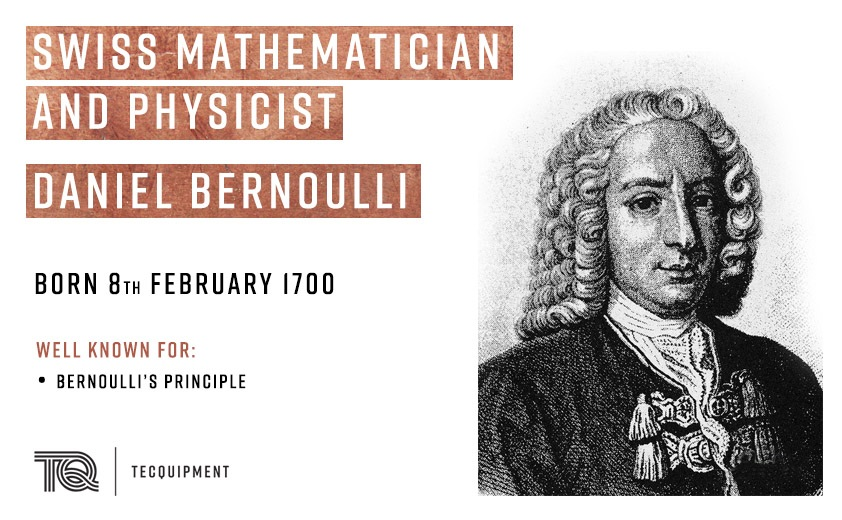# Daniel Bernoulli: Bernoulli’s Principle and Equation

Today we celebrate the contribution that Swiss mathematician and physicist Daniel Bernoulli made to the world of science and engineering, introducing Bernoulli’s principle for understanding fluid mechanics.

Daniel Bernoulli born in the Netherlands 319 years ago studied his passion which was mathematics, and medicine under the guidance of his father Johann Bernoulli who knew all too well from his own experience about the poor pay that the career path would offer.## Bernoulli’s Principle

Bernoulli’s principle states that an increase in the speed of a fluid occurs simultaneously with a decrease in pressure or a decrease in the fluid’s potential energy. Although Bernoulli discovered that pressure decreases when the flow speed increases, it was actually Leonhard Euler who created Bernoulli’s equation.

Bernoulli’s principle can be derived from the principle of conservation of energy. This states in a steady flow, the sum of all forms of energy in a fluid will be the same at all points of that streamline. While all the energy remains constant, an increase in the speed of the fluid will imply there is an increase in the dynamic pressure (kinetic energy). This happens with a simultaneous decrease in the potential energy including the static pressure and internal energy.

## Bernoulli’s Equation

Bernoulli’s equation can be applied to different types of fluid flows which result in various forms of the equation.

The simplest form of Bernoulli’s equation is by using incompressible flow. Incompressible flows are liquids and gases with a low Mach number with the density of the fluid being constant, regardless of the pressure flow.

Bernoulli performed most of his experiments on liquid and used the equation:

Where:

v = Fluid flow speed at a point on a streamline g = Acceleration due to the gravity z = Elevation of the point above a reference plane p = Pressure at the chosen point ρ = Density of the fluid at all points of the fluid

For Bernoulli’s equation to be applied, the following assumptions must be met:

• The flow must be steady. (Velocity, pressure and density cannot change at any point).
• The flow must be incompressible – even when the pressure varies, the density must remain constant along the streamline.
• Friction by viscous forces must be minimal.

## Practical Applications for Learning about Bernoulli’s Principles

• To define Bernoulli’s principle and make direct comparisons from the experiment and theory use TecQuipment’s H5 Bernoulli’s Theorem experiment.
• The AF11 Bernoulli’s Equation experiment is ideal for investigating and supporting Bernoulli’s Equation for use with AF10 Air flow Bench.

## Further Experiments for Bernoulli’s Principles

• Calculate Bernoulli’s equation with the Cavitation in a Venturi unit (H400).
• Show further application to Bernoulli’s equation by experimenting with the Flow Measurement Methods (H10).